# Problem 53730. Easy Sequences 57: "Pretty-gorean" Triangles?

A positive integer x is called a regular number, if and only if there exist a non-negative integer k, such that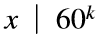. For some reason, such a number is also refered to as an ugly number. Below are the first few regular numbers: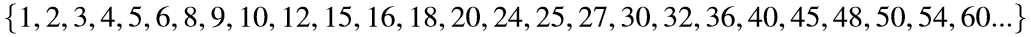Pythagorean triangles are right triangles with all sides having integer lengths. Write a program that counts all Pythagorean triangles whose areas are non-regular numbers and with no sides greater than a given limit s.
Below is the list of all Pythagorean triangles with non-regular areas and with all sides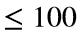:Therefore, for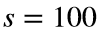, your program output should be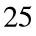.

### Solution Stats

100.0% Correct | 0.0% Incorrect
Last Solution submitted on Aug 23, 2023

### Community Treasure Hunt

Find the treasures in MATLAB Central and discover how the community can help you!

Start Hunting!